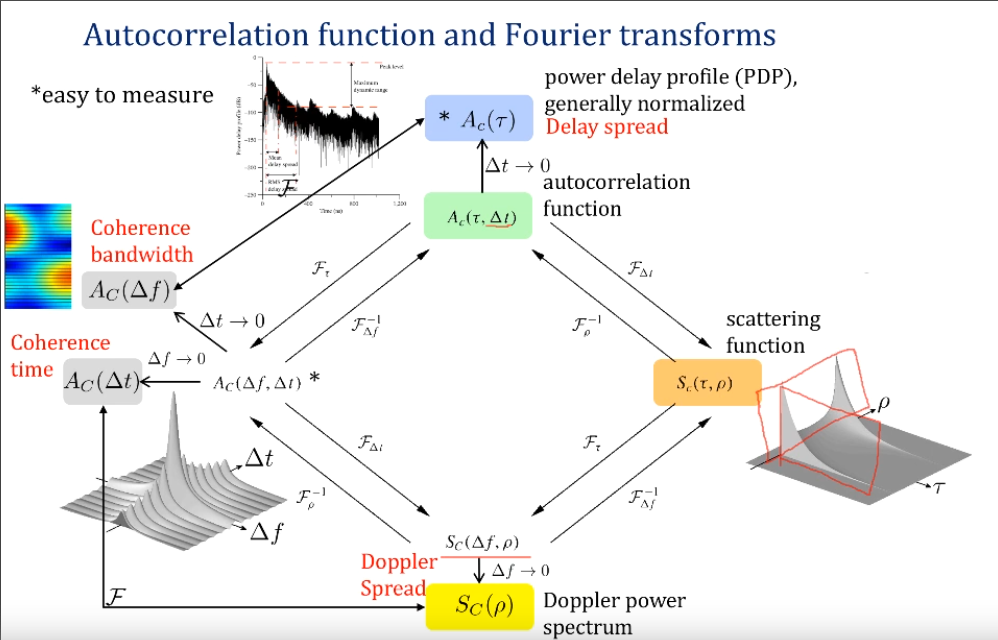# tapped delay line model

TDL is a model for the channel of a wideband fading process.

The channel consists of $$M$$ channel taps, spaced $$\Delta$$ apart such that $$\Delta < \frac{1}{B}$$ where B is the bandwidth.

Each tap is and independent random process following some statistical distribution.

The average power derived from the autocorrelation of $$A_c(\tau, 0)$$, which is also known as the power delay profile.

The correlation of channel at time $$t$$ for two frequencies is simply $$A_C(\Delta f)$$ where $$C$$ is the fourier transform of the channel $$c$$. The autocorrelation of this $$C$$ also gives us coherence time and coherence bandwidth, as well as doppler spread.